# ISO 6976

## Molar Mass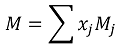Symbol Description Units
M Molar mass of the mixture kg/kmol
Xj Mole fraction of component j -
Mj Molar mass of component j kg/kmol

## Standard Compression Factor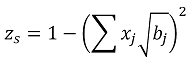Symbol Description Units
zs Standard compression factor -
Xj Mole fraction of component j -
bj Summation factor for component j -

The summation factor for each component (bj) is taken from ISO 6976, Table 2 – Summation factors for components of natural gases at metering reference conditions of 15°C and 101.325 kPa.

## Relative Density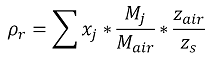Symbol Description Units
ρr Relative density -
Xj Mole fraction of component j -
Mj Molar mass of component j kg/kmol
Zs Standard compression factor -

Constant Description Value Units
zair Compression factor of dry air (1995) 0.99958 -
Mair Molar mass of dry air (1995) 28.9626 kg/kmol
zair Compression factor of dry air (2016) 0.999595 -
Mair Molar mass of dry air (2016) 28.96546 kg/kmol

The molar mass of dry air (Mair) and the compression factor of dry air (zair) are taken from ISO 6976 - 1995 - Annex B or ISO 6976 - 2016 - Annex A Properties of dry air, as appropriate

## Calorific value on a molar basis - 1995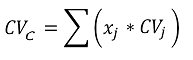Symbol Description Units
CVc Calorific value on molar basis KJ/mol
xj Mole fraction of component j -
CVj Calorific value of component j KJ/mol

The component calorific value (CVj) is taken from ISO 6976 - 1995 - Table 3, Calorific values for components of natural gases at various combustion reference conditions for the ideal gas on a molar basis.

## Calculation of calorific value on a molar basis - 2016

The Superior calorific value is calculated as per 1995 using ISO 6976 - 2016 - Table 3, Gross calorific values on a molar basis for components of natural gas in the ideal gas state at various combustion reference temperatures

The Inferior calorific value is calculated as follows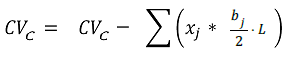Symbol Description Units
CVc Calorific value on molar basis KJ/mol
xj Mole fraction of component j -
bj Number of hydrogen atoms present in each molecule of component j -
L Enthalpy of vaporization of water -

## Calorific Value by Mass - 1995 Definitive and 2016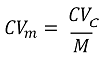Symbol Description Units
CVm Calorific value based on mass MJ/kg
CVc Calorific value on molar basis KJ/mol
M Molar mass of the mixture kg/kmol

## Calorific Value by Mass - 1995 - Alternative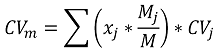Symbol Description Units
CVm Calorific value based on mass MJ/kg
xj Mole fraction of component j -
Mj Molar mass of component j kg/kmol
M Molar mass of the mixture kg/kmol
CVj Calorific value of component j MJ/kg

The component calorific value (CVj) is taken from ISO 6976 - 1995 - Table 4, Calorific values for components of natural gases at various combustion reference conditions for the ideal gas on a mass basis.

## Calorific Value by Volume - 1995 - Definitive and 2016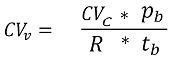Symbol Description Units
CVv Calorific value based on volume MJ/Sm3
CVc Calorific value on molar basis KJ/mol
pb Base pressure bar a
tb Base temperature K

Constant Description Value Units
R Universal Gas Constant 8.314459848484848 J/mol/K

## Calorific Value by Volume - 1995 - Alternative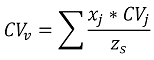Symbol Description Units
CVv Calorific value based on volume MJ/Sm3
xj Mole fraction of component j -
CVj Calorific value of component j MJ/Sm3
Zs Standard compression factor -

The component calorific value (CVj) is taken from ISO 6976 Table 5, Calorific values for components of natural gases at various combustion reference conditions for the ideal gas on a volumetric basis.

# References

• ISO 6976, Natural gas – Calculation of calorific values, density, relative density and Wobbe Index from composition, using table data from 1995 or 2016